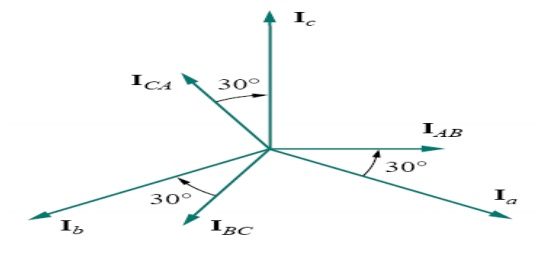Home | | Circuit Theory | Important Short Questions and Answers: Analysing Three Phase Circuits

# Important Short Questions and Answers: Analysing Three Phase Circuits

Electrical and electronics - Circuit Theory - Analysing Three Phase Circuits - Important Short Questions and Answers: Analysing Three Phase Circuits

1.     What are the Advantages of 3 phase system?

i.      Most of the electric power is generated and distributed in three-phase.

ii.     The instantaneous power in a three-phase system can be constant.

iii.     The amount of power, the three-phase system is more economical that the single-phase.

iv.    In fact, the amount of wire required for a three-phase system is less than that required for an equivalent single-phase system.

2.     Define phase, line & neutral?

Phase

Describes or pertains to one element or device in a load, line, or source. It is simply a "branch" of the circuit and could look something like this.

Line

refers to the "transmission line" or wires that connect the source (supply) to the load. It may be modeled as a small impedance (actually 3 of them), or even by just a connecting line.

Neutral

the 4th wire in the 3-phase system. It's where the phases of a Y connection come together. 3. Deifne Phase Voltages & Phase Currents?

Phase Voltages & Phase Currents

the voltages and currents across and through a single branch (phase) of the circuit. Note this definition depends on whether the connection is Wye or Delta!

4. Define line voltage and line current?

Line Currents

the currents flowing in each of the lines (Ia, Ib, and Ic). This definition does not change with connection type.

Line Voltages

the voltages between any two of the lines (Vab, Vbc, and Vca). These may also be referred to as the line-to-line voltages. This definition does not change with connection type.

5. Define line to neutral voltages and line to netural current?

Line to Neutral Voltages

the voltages between any lines and the neutral point (Va, Vb, and Vc). This definition does not change with connection type, but they may not be physically measureable in a Delta circuit.

Line to Neutral Currents

same as the line currents (Ia, Ib, and Ic).

6.       Write the relationship of line and phase voltage and current in star?7. Write the relationship of line and phase voltage and current in delta?8.       Draw the phasor diagram of delta connection?If the voltage source have the same amplitude and frequency ω and are out of phase with each other by 120o, the voltage are said to be balanced.

balanced load is one in which the phase impedances are equal in magnitude and in phase

The load in which the load impedance are not same but having different values. The value of voltage and current are different in each phase.

11.Types of unbalanced load? (JUN-09) a. Unbalanced 3 wire star connected load b. Unbalanced 4 wire star connected load c. Unbalanced 3 wire delta connected load.

12. Write 3 phase power equation?

Apparent power:17. Write the power factor calculation of two wattmeter method?1. Draw two wattmeter methods for measurement of power in 3 phase systems?2.     Comparisons of star and delta connections?

4.     Loads connected in delta dissipate three times more power than when connected in star to the same supply.

5.     For the same power, the phase currents must be the same for both delta and star connections (since power=3I p2Rp), hence the line current in the delta connected system is greater than the line current in the corresponding star-connected system.

6.     To achieve the same phase current in a star-connected system as in a delta-connected system, the line voltage in the star system is √ 3 times the line voltage in the delta system.

7.     Thus for a given power transfer, a delta system is associated with larger line currents (and thus larger conductor cross sectional area) and a star system is associated with a larger line voltage (and thus greater insulation).

3.     Three loads, each of resistance 30 , are connected in star to a 415 V, 3-phase supply. Determine (a) the system phase voltage, (b) the phase current and (c) the line current.

A ‘415 V, 3-phase supply’ means that 415 V is the line voltage, VL

(a) For a star connection, VL =√3Vp Hence phase voltage, Vp = VL/√3

= 415 /√3

= 239.6 V or 240 V

correct to 3 significant figures

(b)             Phase current, Ip = Vp/Rp

= 240/30

= 8 A

(c) For a star connection, Ip = IL Hence the line current, IL = 8 A

Study Material, Lecturing Notes, Assignment, Reference, Wiki description explanation, brief detail
Electrical and electronics : Circuit Theory : Analysing Three Phase Circuits : Important Short Questions and Answers: Analysing Three Phase Circuits |